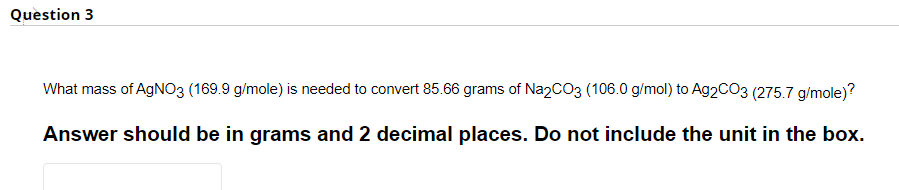Home / Expert Answers / Chemistry / what-mass-of-mathrm-agno-3-169-9-mathrm-g-mathrm-mole-is-needed-to-convert-85-pa923

# (Solved): What mass of $$\mathrm{AgNO}_{3}(169.9 \mathrm{~g} / \mathrm{mole})$$ is needed to convert $$85 ...What mass of \( \mathrm{AgNO}_{3}(169.9 \mathrm{~g} / \mathrm{mole})$$ is needed to convert $$85.66$$ grams of $$\mathrm{Na}_{2} \mathrm{CO}_{3}(106.0 \mathrm{~g} / \mathrm{mol})$$ to $$\mathrm{Ag}_{2} \mathrm{CO}_{3}(275.7 \mathrm{~g} / \mathrm{mole})$$ ? Answer should be in grams and 2 decimal places. Do not include the unit in the box.

We have an Answer from Expert